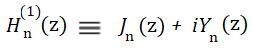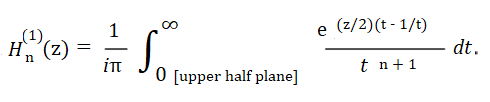# Hankel Function

The Hankel function is a complex-valued solution to Bessel’s differential equation. These functions are very useful for problems involving spherical and cylindrical wave propagation.

It is also called a Bessel function of the third kind, or a Weber Function.

In essence, a Hankel Function is a combination of Bessel functions of the first kind and second kind. So you can think of it as a “type” of Bessel function. In many mathematical programs, the Hankel is defined in terms of the Bessel. For example, in MATLAB, the Hankel function syntax is:

• First Kind: H = besselh(nu,Z),
• First or Second Kind: H = besselh(nu,K,Z).

Where:

• k = each element (1 or 2) of the complex array z,
• nu = the order of the Hankel function. This must be the same size as Z, or one can be scalar. For example, besselh(4,Z).

As far as evaluating the function, you’re probably going to want to use software, because it is notoriously difficult to evaluate numerically by hand, especially for large order and large argument (Jentschura, 2011).

## Hankel Function of the First Kind

The Hankel function of the first kind is defined as:Where:

• Jn(z) = Bessel function of the first kind,
• Yn(z) = Bessel function of the second kind.

## Contour Integral Definition

The Hankel function can also be represented by the following contour integral:## Real Life Applications

Bessel function theory (and the associated Hankel functions) have many real life applications, including:

• Acoustics,
• Atomic and nuclear physics,
• Hydrodynamics,

## References

Arfken, G. Hankel Functions. Mathematical Methods for Physicists, 3rd ed. Orlando, FL: Academic Press, pp. 604-610, 1985.
Jentschura, U. Numerical calculation of Bessel, Hankel and Airy functions. Retrieved November 30, 2019 from: http://arxiv-export-lb.library.cornell.edu/pdf/1112.0072
Korenev, B. (2002). Bessel Functions and Their Applications. CRC Press.
Mathworks. Besselh. Retrieved November 30 from: https://www.mathworks.com/help/matlab/ref/besselh.html
Morse, P. M. and Feshbach, H. Methods of Theoretical Physics, Part I. New York: McGraw-Hill, pp. 623-624, 1953.

CITE THIS AS:
Stephanie Glen. "Hankel Function" From StatisticsHowTo.com: Elementary Statistics for the rest of us! https://www.statisticshowto.com/hankel-function/